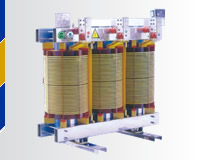The capacity is unit of transformer power, the symbol is KVA. The nameplate of transformer must label capacity. But when we need to install or increase capacity, how to make sure the capacity of transformer?
The first step to calculate the load capacity of transformer. For the steady power distribution system, the load capacity could reach 85%. That means the transformer power could reach 85% rated capacity. We need consider the volatility of transformer when they work. They exist ups and downs. So the load capacity need to be 60% to 70%. We choose high capacity.
The matters need attention when we choose transformer.
1, to know the voltage of equipment, the actual power and condition of users. The capacity of transformer need to match electric equipment power, character and service time.
2, when the transformer works, the electric load need to reach 75%-90% rated capacity. If the actual load is less than 50% when they works, you need to change the small capacity transformer.
3 When selecting the capacity of the transformer, the input voltage value of the transformer is determined according to the line. According to the selection of the output voltage value by the electric equipment, it is better to select the three-phase four-wire power supply, so that the industrial power and the lighting power can be provided at the same time.
4. The determination of the transformer capacity should accurately count all the power loads, consider the power characteristics, and choose the appropriate transformer capacity. If the transformer capacity is too large, it will increase the basic electricity cost and increase the loss. If the capacity is too small, it will easily trip and heat up.